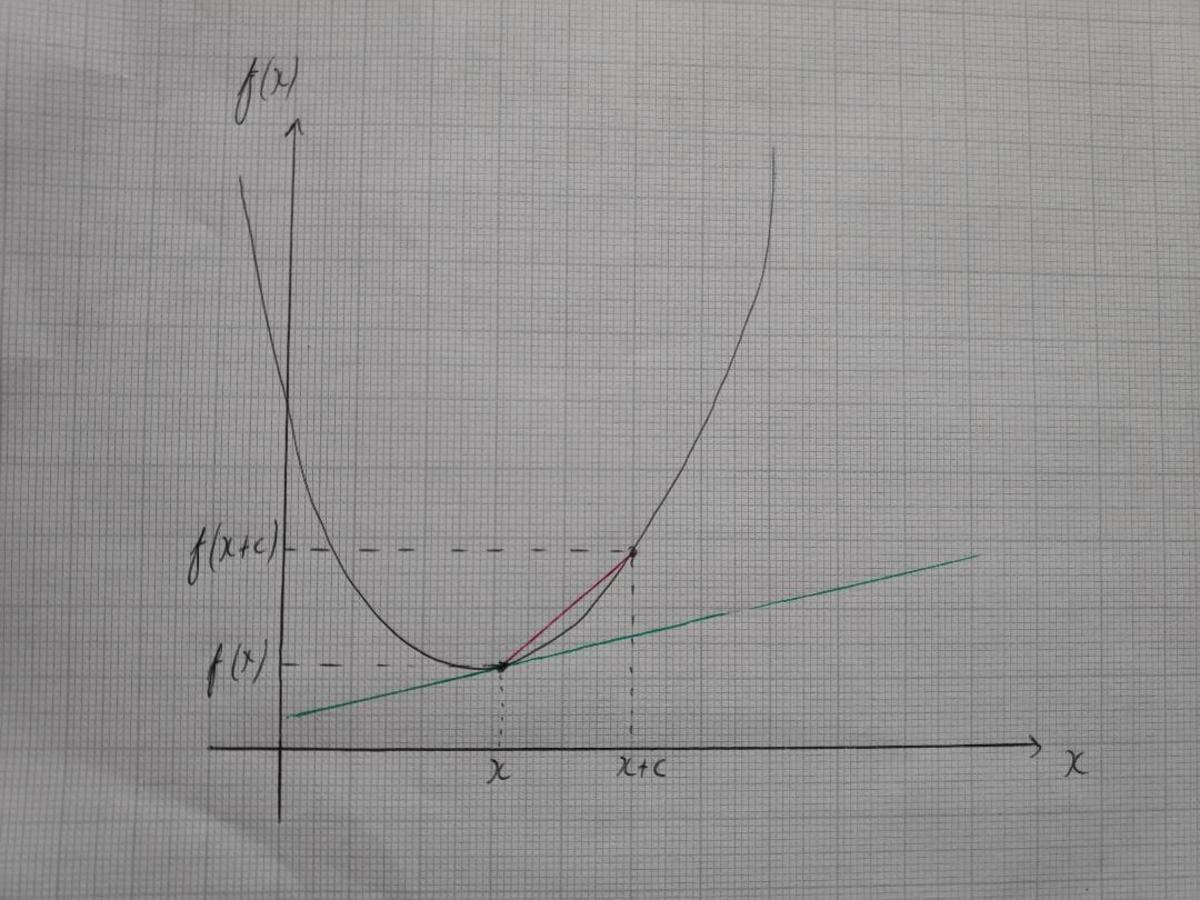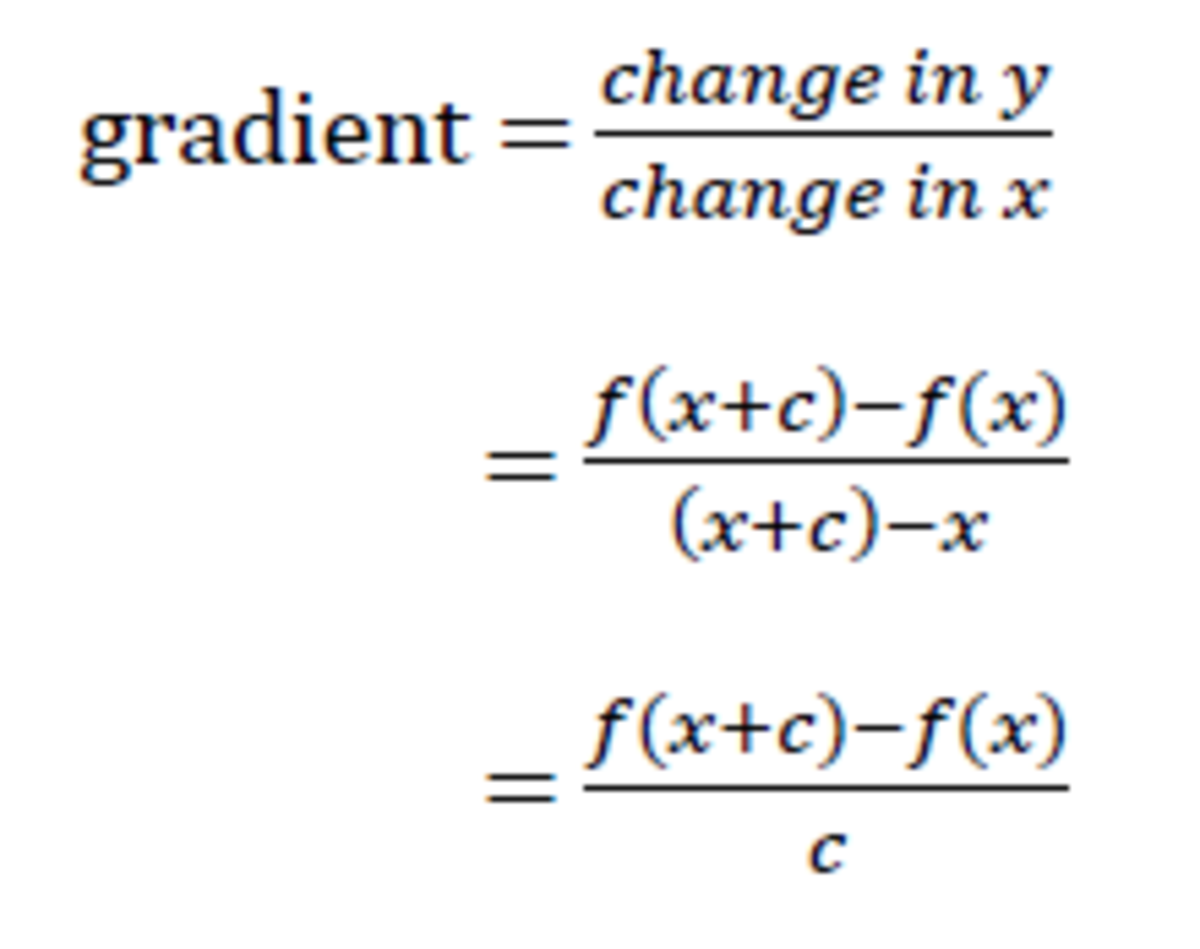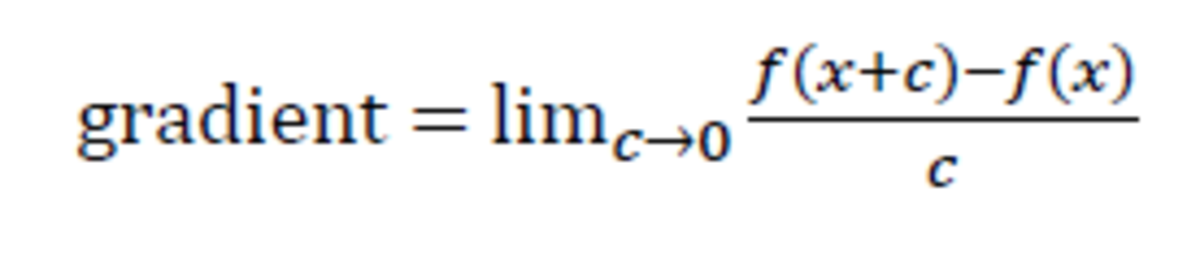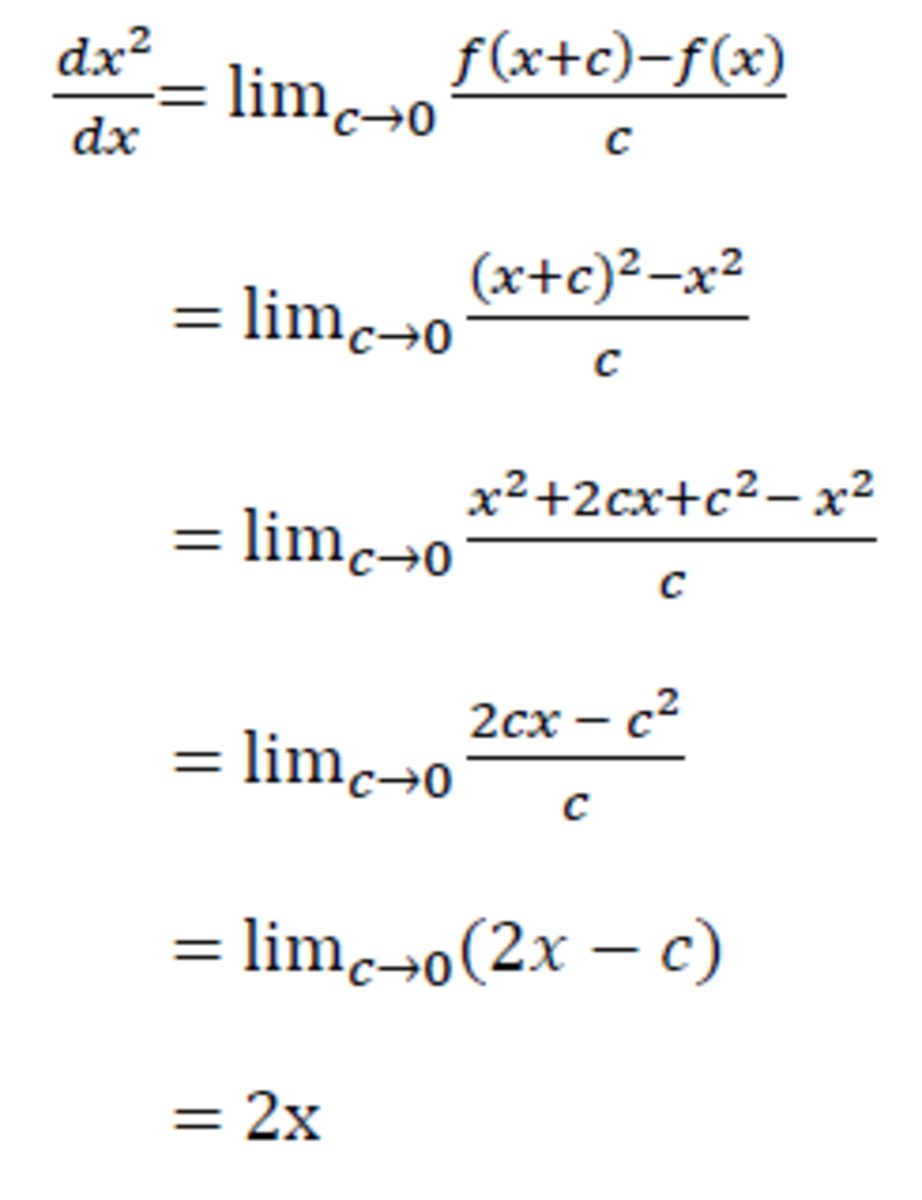# How to Differentiate From First Principles

• Author:
• Updated date:

I am a former maths teacher and owner of DoingMaths. I love writing about maths, its applications, and fun mathematical facts.Isaac Newton (1642 - 1726)Public Domain

## What Is Differentiation?

Differentiation is used to find the rate of change of a mathematical function as its input changes. For example, by finding the rate of change of an object's velocity, you get its acceleration; by finding the rate of change of a function on a graph, you find its gradient.

Discovered independently by the British mathematician Issac Newton and the German mathematician Gottfried Leibnitz in the late 17th century (we still use Leibnitz's notation to this day), differentiation is an extremely useful tool in mathematics, physics and much more. In this article, we look at how differentiation works and how to differentiate a function from first principles.A Curved Line With Its Gradient Marked OnDavid Wilson

## Differentiating From First Principles

Assume you have a function f(x) on a graph, as in the picture above, and you want to find the gradient of the curve at the point x (the gradient is shown in the picture by the green line). We can find an approximation to the gradient by choosing another point further along the x-axis, which we will call x + c (our original point plus a distance of c along the x-axis).

By joining these points, we get a straight line (in red on our diagram). We can find the gradient of this red line by finding the change in y divided by the change in x.

The change in y is f(x+c) - f(c) and the change in x is (x+c) - x. Using these, we get the following equation:The change in y is f(x+c) - f(c) and the change in x is (x+c) - x.David Wilson
Scroll to Continue

## Read More From Owlcation

So far, all we have is a very rough approximation of the gradient of our line. You can see from the diagram that the red approximate gradient is significantly steeper than the green gradient line. If we reduce c, however, we move our second point closer to the point (x, f(x)), and our red line gets closer and closer to having the same gradient as f(x).

Reducing c obviously reaches a limit when c=0, making x and x+c the same point. However, our formula for the gradient has c for a denominator and so is undefined when c=0 (because we can't divide by 0). To get around this, we want to find out the limit of our formula as c→0 (as c tends towards 0). Mathematically, we write this as it is shown in the image below.Gradient defined by its limit as C tends towards zeroDavid Wilson

## Using Our Formula to Differentiate a Function

We now have a formula that we can use to differentiate a function by first principles. Let's try it out with an easy example; f(x) = x2. In this example, I have used the standard notation for differentiation; for the equation y = x2, we write the derivative as dy/dx or, in this case (using the right hand side of the equation), dx2/dx.

Note: When using f(x) notation, it is standard to write the derivative of f(x) as f ' (x). If this was differentiated again, we would get f '' (x) and so on.How to differentiate x^2 by first principles

## Differentiating Further Functions

So there we have it. If you have a line with the equation y=x2, the gradient can be calculated at any point using the equation dy/dx = 2x. e.g. at the point (3,9), the gradient would be dy/dx = 2×3 = 6.

We can use this exact same method of differentiation by first principles to differentiate further functions such as x5, sin x, etc. Try using what we have done in this article to differentiate these two. Hint: the method for y = x5 is very similar to that used for y = x. The method for y = sin x is a little trickier and requires some trigonometric identities, but the maths used shouldn't need to go beyond the A-Level standard.

This content is accurate and true to the best of the author’s knowledge and is not meant to substitute for formal and individualized advice from a qualified professional.